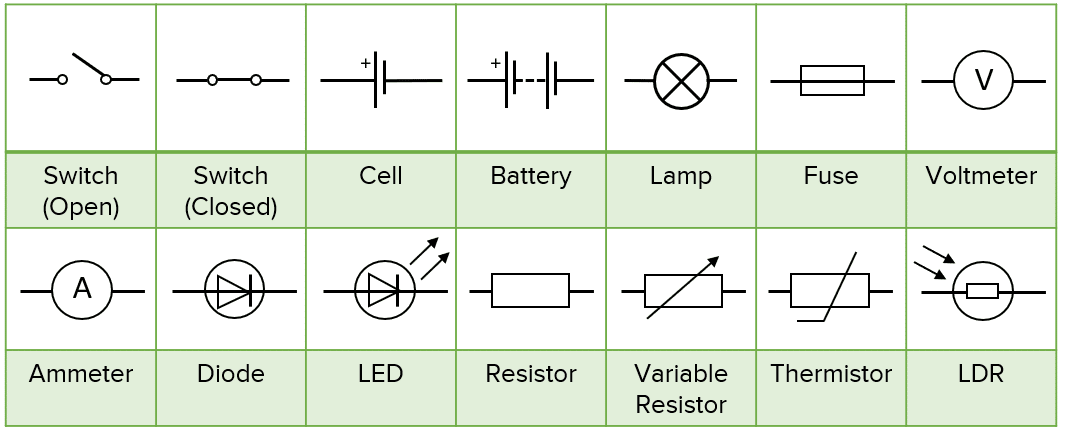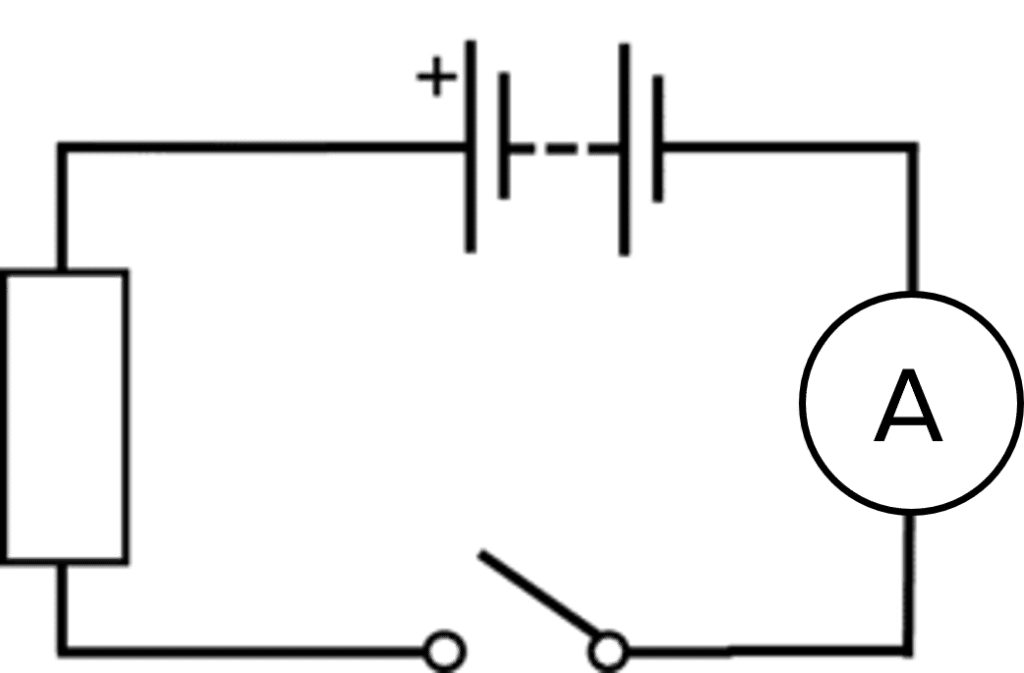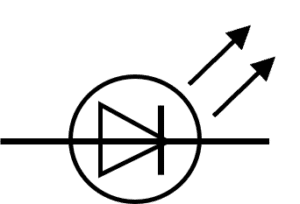# Electrical Charge and Current

GCSEAQACombined Science FoundationCombined Science HigherPhysics FoundationPhysics Higher

## Electrical Charge and Current

Electric current flows around circuits to power circuit components such as lamps, motors and buzzers.

## Circuit Components

Circuits can be made up of a combination of many different circuit components. Different circuit components have different properties and functions

In a circuit diagram, we use specific symbols called circuit symbols to represent each component.

You need to be able to draw and recognise the following circuit symbolsGCSECombined Science FoundationCombined Science HigherPhysics FoundationPhysics HigherAQA

## Electrical Current

Electric current is a flow of electrical charge. Electrical charge is measured in Coulombs $\text{(C)}$ and current is measured in amperes $\text{(A)}$. The current can be thought of as the rate of flow of charge around the circuit. You can measure current using an ammeter. You can calculate the charge that has flown around a circuit in a certain time $\text{(s)}$ using the following equation:

$\color{f21cc2}{Q=It}$

• $Q=$ charge flow in joules $\text{(J)}$
• $I=$ current in amps $\text{(A)}$
• $t=$ time in seconds $\text{(s)}$

Remember that current will only flow through a circuit when it makes a complete loop

GCSECombined Science FoundationCombined Science HigherPhysics FoundationPhysics HigherAQA

## Example: Calculating ChargeA student connects a battery to an ammeter and a resistor as shown in the diagram. The student closes the switch for $1\text{ minute}$ and then opens it again. During this minute, the ammeter reads $2.5\text{ A}$

Calculate the charge that flowed through the circuit during the time that the switch was closed.

[3 marks]

$t= \textcolor{f21cc2}{1 \text{ minute}}=60\text{ s} \\\bold{Q=It}=\textcolor{7cb447}{2.5} \times60=\bold{150 \, C}$
GCSECombined Science FoundationCombined Science HigherPhysics FoundationPhysics HigherAQA

## Electrical Charge and Current Example QuestionsGold Standard Education

Variable resistorGold Standard Education

The flow of electrical chargeGold Standard Education

$\bold{Q=It}=4\text{A}\times 10\text{ s}=\bold{40\text{ C}}$Gold Standard EducationProduct